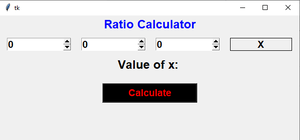# Ratio Calculator GUI using Tkinter

• Last Updated : 09 Nov, 2021

Prerequisite: Python GUI – tkinter

Tkinter is the most commonly used library for developing GUI (Graphical User Interface) in Python. It is a standard Python interface to the Tk GUI toolkit shipped with Python. As Tk and Tkinter are available on most of the Unix platforms as well as on the Windows system, developing GUI applications with Tkinter becomes the fastest and easiest.

In this article, we are going to discuss how to create a ratio calculator using Tkinter module.

## What is the Ratio Calculator?

It is the calculator that computes the denominator base on the given ratio values.

Explanation:

```Input:
a = 10
b = 20
c = 30
d = ?
a : d = c : d
Output:
value of d is 60.0```

Below is how the calculator will look like:Formula Used:

`a/b = c/d`

Here a and b are given ratio and c is entered value for which we have to find the ratio i.e. the denominator d.

Step-by-step Approach:

• Create Normal Tkinter Window

## Python3

 `# Import module``from` `tkinter ``import` `*` `# Create object``root ``=` `Tk()` `# Adjust size``root.geometry(``"400x400"``)` `# Execute tkinter``root.mainloop()`

Output:• Create One Frame using Frame() method and add three spin box using Spinbox() method.

Syntax:

```# Create Frame
frame = Frame(Object Name)

# Create Spin Box
# Enter Range
Spinbox(frame, from_= 0, to = 10,**attr)```
• Create Another Frame, add Button, Label and create a function called as ratio_calculator, which will calculate the value of X.

Syntax:

```# Create Frame
frame1 = Frame(Object Name)

# Create Label
Label(frame1, text="Enter Text",**attr)

# Create Button
Button(root,text="Enter Text",**attr)```

Below is the implementation:

## Python3

 `# Import Module``from` `tkinter ``import` `*` `# Create Object``root ``=` `Tk()` `# Set height and width``width ``=` `600``height ``=` `250` `# Set Geometry and min, max size``root.geometry(f``"{width}x{height}"``)``root.maxsize(width, height)``root.minsize(width, height)` `# Create Label``Label(root, text``=``"Ratio Calculator"``, font``=``(``    ``"Helvetica"``, ``18``, ``"bold"``), fg``=``"blue"``).pack()`  `# Function will calculate the value of x``def` `ratio_calculator():``        ``# Get the value of spinbox using get() method``    ``s11 ``=` `int``(s1.get())``    ``s22 ``=` `int``(s2.get())``    ``s33 ``=` `int``(s3.get())` `    ``# Formula Used``    ``value ``=` `(s33``*``s22)``/``s11` `    ``# change the text of label using config method``    ``value_of_x.config(text``=``value)`  `# Create Frame``frame ``=` `Frame(root)``frame.pack()` `# Create Spin Boxes``s1 ``=` `Spinbox(frame, from_``=``0``, to``=``10000000``, width``=``10``,``             ``font``=``(``"Helvetica"``, ``14``, ``"bold"``))``s1.pack(side``=``LEFT, padx``=``10``, pady``=``10``)``s2 ``=` `Spinbox(frame, from_``=``0``, to``=``10000000``, width``=``10``,``             ``font``=``(``"Helvetica"``, ``14``, ``"bold"``))``s2.pack(side``=``LEFT, padx``=``10``, pady``=``10``)``s3 ``=` `Spinbox(frame, from_``=``0``, to``=``10000000``, width``=``10``,``             ``font``=``(``"Helvetica"``, ``14``, ``"bold"``))``s3.pack(side``=``LEFT, padx``=``10``, pady``=``10``)` `# Add Another Label``Label(frame, text``=``"X"``, width``=``10``, font``=``(``"Helvetica"``,``                                       ``14``, ``"bold"``),``      ``borderwidth``=``1``, relief``=``"solid"``).pack(side``=``LEFT,``                                          ``padx``=``10``,``                                          ``pady``=``10``)` `# Add Another Frame``frame1 ``=` `Frame(root)``frame1.pack()` `x_value ``=` `Label(frame1, text``=``"Value of x:"``,``                ``font``=``(``"Helvetica"``, ``18``, ``"bold"``))``x_value.pack(side``=``LEFT)` `value_of_x ``=` `Label(frame1, text``=``"",``                   ``font``=``(``"Helvetica"``, ``18``, ``"bold"``))``value_of_x.pack(side``=``LEFT)` `# Create Button``Button(root, text``=``"Calculate"``, borderwidth``=``2``, width``=``15``,``       ``font``=``(``"Helvetica"``, ``14``, ``"bold"``),``       ``command``=``ratio_calculator, fg``=``"red"``,``       ``bg``=``"black"``).pack(pady``=``20``)` `# Execute Tkinter``root.mainloop()`

Output:

My Personal Notes arrow_drop_up